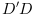Scilab 6.0.0
Change language to:
English - Français - 日本語 - Português -

See the recommended documentation of this function

# lqr

LQ compensator (full state)

### Syntax

`[K,X]=lqr(P12)`
`[K,X]=lqr(P,Q,R [,S])`

### Arguments

P12

A state space representation of a linear dynamical system (see syslin)

P

A state space representation of a linear dynamical system (see syslin)

Q

Real symmetric matrix, with same dimensions as P.A

R

full rank real symmetric matrix

S

real matrix, the default value is `zeros(size(R,1),size(Q,2))`

K

a real matrix, the optimal gain

X

a real symmetric matrix, the stabilizing solution of the Riccati equation

### Description

Syntax `[K,X]=lqr(P)`

Computes the linear optimal LQ full-state gain K for the state space representation PAnd instantaneous cost function in l2-norm:Syntax `[K,X]=lqr(P,Q,R [,S])`

Computes the linear optimal LQ full-state gain K for the linear dynamical system P:And instantaneous cost function in l2-norm:### Remark

In this case the P.C and P.D componants of the system are ignored

### Algorithm

• For a continuous plant, ifis the stabilizing solution of the Riccati equation:the linear optimal LQ full-state gain K is given by• For a discrete plant, ifis the stabilizing solution of the Riccati equation:the linear optimal LQ full-state gain K is given byAn equivalent form for the equation iswithThe gain `K` is such thatis stable.

The resolution of the Riccati equation is obtained by schur factorization of the 3-blocks matrix pencils associated with these Riccati equations:

• For a continuous plant• For a discrete time plant### Caution

It is assumed that matrixoris non singular.

### Remark

If the full state of the system is not available, An estimator can be built using the lqe or the lqg function.

### Examples

Assume the dynamical system formed by two masses connected by a spring and a damper:A force(whereis a noise) is applied to the big one. Here it is assumed that the deviations from equilibrium positions of the massandpositions has well as their derivatives are measured.

A state space representation of this system is:The LQ cost is defined byThe following instructions may be used to compute a LQ compensator of this dynamical system.

```// Form the state space model (assume full state output)
M = 1; m = 0.2; k = 0.1; b = 0.004;
A = [  0     1    0    0
-k/M  -b/M  k/M  b/M
0     0    0    1
k/m  b/m  -k/m  -b/m];
B = [0; 1/M; 0; 0];
C = eye(4,4);
P = syslin("c",A, B, C);
//The compensator weights
Q_xx=diag([15 0 3 0]); //Weights on states
R_uu   = 0.5; //Weight on input
Kc=lqr(P,Q_xx,R_uu);

//form the Plant+compensator system

C=[1 0 0 0  //dy1
0 0 1 0];//dy2
S=C*(P/.(-Kc));
//check system stability
and(real(spec(S.A))<0)
// Check by simulation
dt=0.1;
t=0:dt:30;
u=0.1*rand(t);
y=csim(u,t,S,[1;0;0;0]);
clf;plot(t',y');xlabel(_("time (s)"))
L=legend(["\$dy_1\$","\$dy_2\$"]);L.font_size=4;```### Reference

Engineering and Scientific Computing with Scilab, Claude Gomez and al.,Springer Science+Business Media, LLC,1999, ISNB:978-1-4612-7204-5